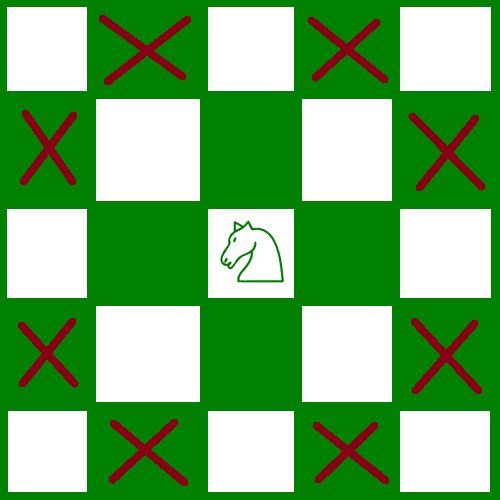Open in App
Not now

# Maximum non-attacking Knights that can be placed on an N*M Chessboard

• Difficulty Level : Medium
• Last Updated : 17 Sep, 2019

Given an N*M chessboard. The task is to find the maximum number of knights that can be placed on the given chessboard such that no knight attack some other knight.

Example

Input: N = 1, M = 4
Output: 4
Place a knight on every cell of the chessboard.

Input: N = 4, M = 5
Output: 10

## Recommended: Please try your approach on {IDE} first, before moving on to the solution.

Approach: As we know that a knight can attack in two ways. Here are the places which he can attack.Here, in the picture, the knight is on white color and attacks only the black color. Thus. we concluded that a knight can attack only on a different color.
We can take help of this fact and use it for our purpose. Now as we know knight attacks on different color so we can keep all knights on the same color i.e. all on white or all on black. Thus making the highest number of knights which can be placed.
To find the number of black or white, it is simply half of the total blocks on board.

Total Blocks = n * m
Blocks of the same color = (n * m) / 2

Corner cases:

• If there is only a single row or column. Then all the blocks can be filled by knights as a knight cannot attack in the same row or column.• If there are only two rows or columns. Then every two columns (or rows) will be filled with knights and every consecutive two columns (or rows) will remain empty. As demonstrated in the picture.• Below is the implementation of the above approach:

## C++

 `// C++ implementation of the approach``#include ``using` `namespace` `std;`` ` `// Function to return the maximum number of``// knights that can be placed on the given``// chessboard such that no two``// knights attack each other``int` `max_knight(``int` `n, ``int` `m)``{`` ` `    ``// Check for corner case #1``    ``// If row or column is 1``    ``if` `(m == 1 || n == 1) {`` ` `        ``// If yes, then simply print total blocks``        ``// which will be the max of row or column``        ``return` `max(m, n);``    ``}`` ` `    ``// Check for corner case #2``    ``// If row or column is 2``    ``else` `if` `(m == 2 || n == 2) {`` ` `        ``// If yes, then simply calculate``        ``// consecutive 2 rows or columns``        ``int` `c = 0;``        ``c = (max(m, n) / 4) * 4;`` ` `        ``if` `(max(m, n) % 4 == 1) {``            ``c += 2;``        ``}``        ``else` `if` `(max(m, n) % 4 > 1) {``            ``c += 4;``        ``}``        ``return` `c;``    ``}`` ` `    ``// For general case, just print the``    ``// half of total blocks``    ``else` `{``        ``return` `(((m * n) + 1) / 2);``    ``}``}`` ` `// Driver code``int` `main()``{``    ``int` `n = 4, m = 5;`` ` `    ``cout << max_knight(n, m);`` ` `    ``return` `0;``}`

## Java

 `// Java implementation of the approach ``import` `java.io.*;`` ` `class` `GFG ``{``     ` `// Function to return the maximum number of ``// knights that can be placed on the given ``// chessboard such that no two ``// knights attack each other ``static` `int` `max_knight(``int` `n, ``int` `m) ``{ `` ` `    ``// Check for corner case #1 ``    ``// If row or column is 1 ``    ``if` `(m == ``1` `|| n == ``1``) ``    ``{ `` ` `        ``// If yes, then simply print total blocks ``        ``// which will be the max of row or column ``        ``return` `Math.max(m, n); ``    ``} `` ` `    ``// Check for corner case #2 ``    ``// If row or column is 2 ``    ``else` `if` `(m == ``2` `|| n == ``2``) ``    ``{ `` ` `        ``// If yes, then simply calculate ``        ``// consecutive 2 rows or columns ``        ``int` `c = ``0``; ``        ``c = (Math.max(m, n) / ``4``) * ``4``; `` ` `        ``if` `(Math.max(m, n) % ``4` `== ``1``)``        ``{ ``            ``c += ``2``; ``        ``} ``        ``else` `if` `(Math.max(m, n) % ``4` `> ``1``) ``        ``{ ``            ``c += ``4``; ``        ``} ``        ``return` `c; ``    ``} `` ` `    ``// For general case, just print the ``    ``// half of total blocks ``    ``else` `    ``{ ``        ``return` `(((m * n) + ``1``) / ``2``); ``    ``} ``} `` ` `// Driver code ``public` `static` `void` `main (String[] args) ``{``    ``int` `n = ``4``, m = ``5``; ``    ``System.out.println (max_knight(n, m)); ``}``}`` ` `// This code is contributed by ajit `

## Python3

 `# Python3 implementation of the approach `` ` `# Function to return the maximum number of ``# knights that can be placed on the given ``# chessboard such that no two ``# knights attack each other ``def` `max_knight(n, m) : `` ` `    ``# Check for corner case #1 ``    ``# If row or column is 1 ``    ``if` `(m ``=``=` `1` `or` `n ``=``=` `1``) :`` ` `        ``# If yes, then simply print total blocks ``        ``# which will be the max of row or column ``        ``return` `max``(m, n); `` ` `    ``# Check for corner case #2 ``    ``# If row or column is 2 ``    ``elif` `(m ``=``=` `2` `or` `n ``=``=` `2``) :`` ` `        ``# If yes, then simply calculate ``        ``# consecutive 2 rows or columns ``        ``c ``=` `0``; ``        ``c ``=` `(``max``(m, n) ``/``/` `4``) ``*` `4``; `` ` `        ``if` `(``max``(m, n) ``%` `4` `=``=` `1``) :``            ``c ``+``=` `2``; ``         ` `        ``elif` `(``max``(m, n) ``%` `4` `> ``1``) :``            ``c ``+``=` `4``; `` ` `        ``return` `c; `` ` `    ``# For general case, just print the ``    ``# half of total blocks ``    ``else` `:``        ``return` `(((m ``*` `n) ``+` `1``) ``/``/` `2``); `` ` `# Driver code ``if` `__name__ ``=``=` `"__main__"` `: `` ` `    ``n ``=` `4``; m ``=` `5``; `` ` `    ``print``(max_knight(n, m)); `` ` `# This code is contributed by AnkitRai01`

## C#

 `// C# implementation of the approach ``using` `System;`` ` `class` `GFG``{``         ` `// Function to return the maximum number of ``// knights that can be placed on the given ``// chessboard such that no two ``// knights attack each other ``static` `int` `max_knight(``int` `n, ``int` `m) ``{ `` ` `    ``// Check for corner case #1 ``    ``// If row or column is 1 ``    ``if` `(m == 1 || n == 1) ``    ``{ `` ` `        ``// If yes, then simply print total blocks ``        ``// which will be the max of row or column ``        ``return` `Math.Max(m, n); ``    ``} `` ` `    ``// Check for corner case #2 ``    ``// If row or column is 2 ``    ``else` `if` `(m == 2 || n == 2) ``    ``{ `` ` `        ``// If yes, then simply calculate ``        ``// consecutive 2 rows or columns ``        ``int` `c = 0; ``        ``c = (Math.Max(m, n) / 4) * 4; `` ` `        ``if` `(Math.Max(m, n) % 4 == 1)``        ``{ ``            ``c += 2; ``        ``} ``        ``else` `if` `(Math.Max(m, n) % 4 > 1) ``        ``{ ``            ``c += 4; ``        ``} ``        ``return` `c; ``    ``} `` ` `    ``// For general case, just print the ``    ``// half of total blocks ``    ``else``    ``{ ``        ``return` `(((m * n) + 1) / 2); ``    ``} ``} `` ` `// Driver code ``static` `public` `void` `Main ()``{``    ``int` `n = 4, m = 5; ``    ``Console.Write(max_knight(n, m)); ``}``}`` ` `// This code is contributed by Tushil.`

Output:

```10
```

My Personal Notes arrow_drop_up